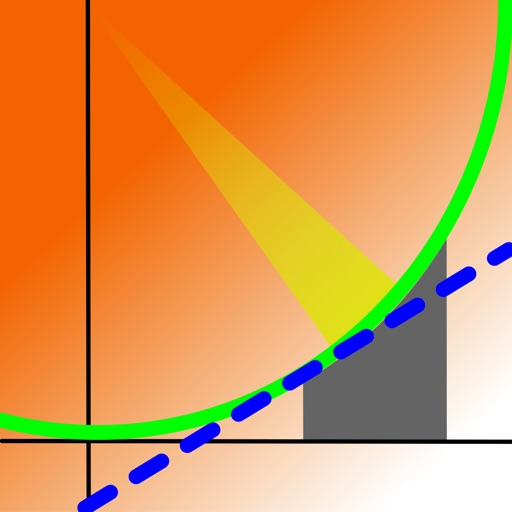## V-Solve has all of the features of V-Graph Save, except for graphing and the ability to save equations to the library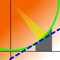# V-Solve

by Staghorn Ridge Software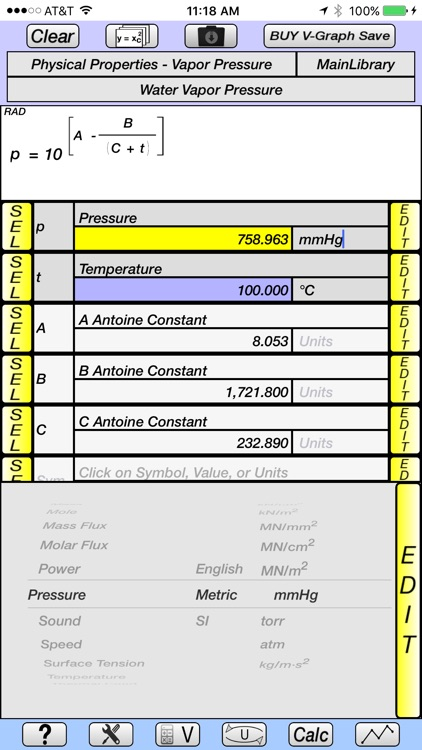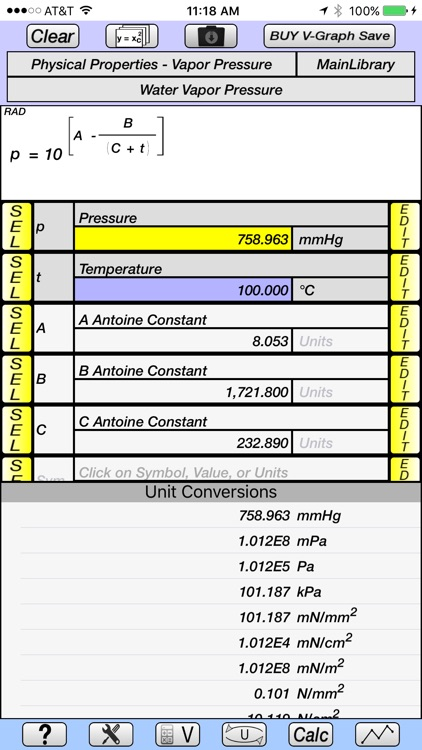V-Solve has all of the features of V-Graph Save, except for graphing and the ability to save equations to the library. V-Solve has a demo feature which shows the graphing features of V-Graph Save for a select set of equations.### App Details

Version
1.4
Rating
(2)
Size
9Mb
Genre
Utilities Productivity
Last updated
February 15, 2021
Release date
February 2, 2017

### App Screenshots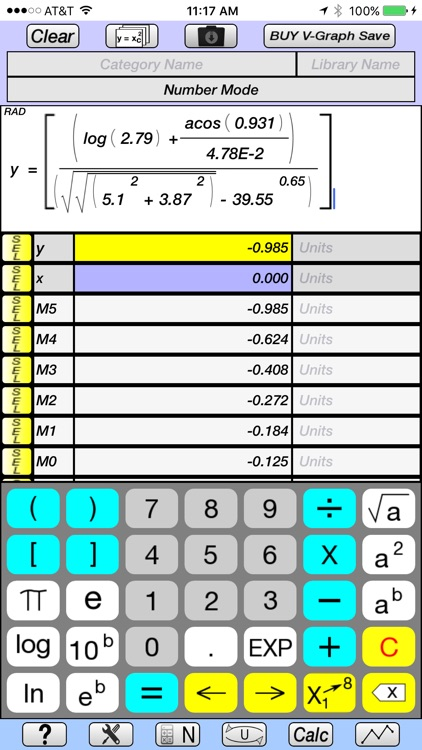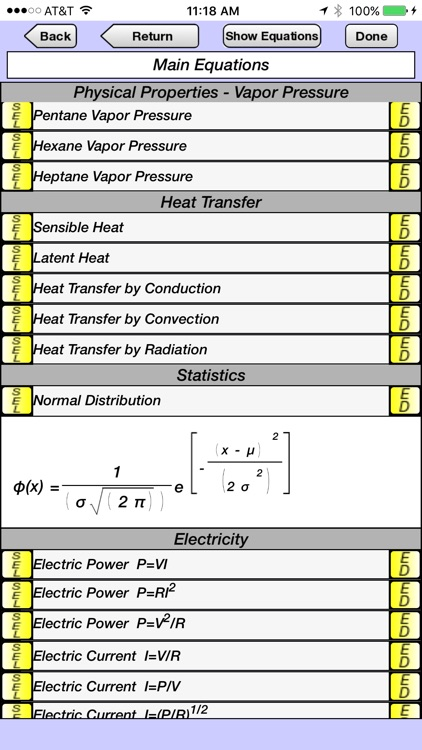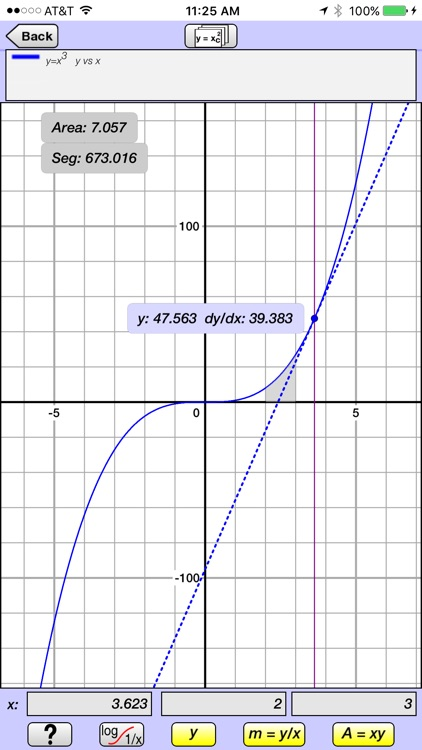### App Store Description

V-Solve has all of the features of V-Graph Save, except for graphing and the ability to save equations to the library. V-Solve has a demo feature which shows the graphing features of V-Graph Save for a select set of equations.

Overview of Features

• Solves explicit and implicit equations.
• Equations are entered and displayed in their natural form, as they would appear in a textbook.
• 59 categories of units in library, divided into english, metric, and SI systems.
• Constants and user-defined unit conversions can be saved into libraries.
• Well-organized Main, Trigonometric, English, and Greek keypads.
• Help screen overlay provides pop-up description of the function of key buttons.
• Tutorials provide demonstration of the calculator’s capabilities by programmatically stepping through equation creation and unit conversion examples.

Equation Creation and Display

• Equations are created and displayed in their natural form, as they would appear in a textbook.
• Number Mode allows equations based on y (dependent variable) and x (independent variable) to be quickly created, solved, and have unit conversions carried out on the dependent variable.
• Variable Mode allows equations with more complex variables to be created:
• Unit conversions can be carried out on result.
• Super- and subscripting of variables.
• Functions include square root, squared, x to any power, log, ln, 10 to any power, e to any power, factorial, trigonometric (including inverse), hyperbolic (including inverse).
• Button selector allows the variable symbol or its value to be displayed in the equation.
• Previously used equations are maintained in memory and can be re-accessed by means of a drop-down selection window.

Unit Conversions

• Unit conversions are made simultaneously, and are displayed as a table of all units of the same category.
• New units can be created and saved to the library, using the base units (m, s, g, ft, lb, etc).
• New units are easily created using picker dials for the metric prefix (k, M, G, etc), base units, and power of 1, 2, 3, etc.

Constant Libraries

• Physics constant library.
Constants can be saved to libraries under user-defined categories.

Other Features

• E-mail contact is accessed through a button provided in the Help/Tutorial screens.
• Memory stack maintains values, variable symbols, and equation names of an unlimited number of previous calculations, which can be easily accessed for future calculations.
• Multiple answers for implicit equations are saved to the memory stack.
• Period or comma can be used as decimal separator.
• Degrees or radians for trigonometric functions.
• Decimal or Scientific Notation, and number of significant digits can be easily specified.
Convergence criteria and maximum number of iterations can be specified for implicit equations.